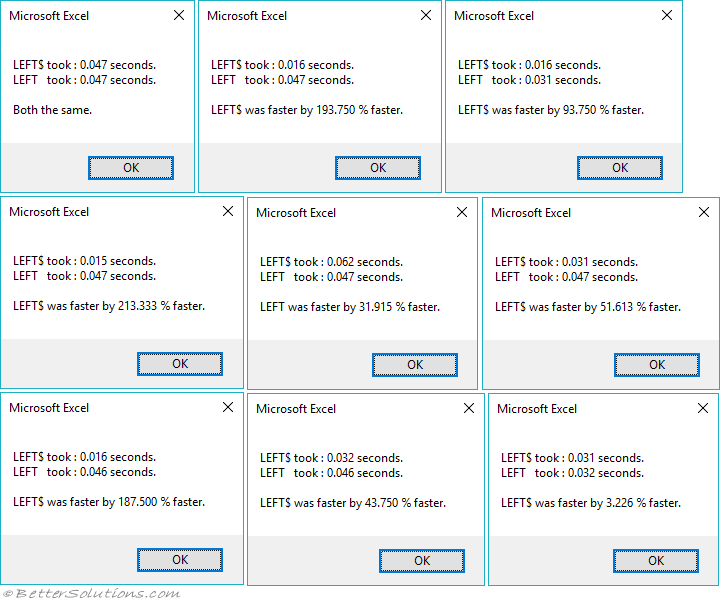# Speed Test

### Which Is Faster ?

There are rumours that the Dollar Functions are faster but this is really not worth worrying about.
There is definitely a difference in speed when you are iterating through a very large number of times.
Unless you are iterating through hundreds of thousands of times, there is no difference.

`Declare PtrSafe Function GetTickCount Lib "kernel32.dll" () As Long Sub TimingSubroutine() Dim iloop As Integer Dim lRow As Long Dim dbTime As Double Dim sShort As String Dim left_nodollar As Single Dim left_dollar As Single Dim percentage As Single         For iloop = 1 To 10         dbTime = GetTickCount()         For lRow = 1 To 300000   '300,000 times              sShort = Left\$("some text", 4)         Next lRow         left_dollar = (GetTickCount() - dbTime) / 1000                 dbTime = GetTickCount()         For lRow = 1 To 300000   '300,000 times              sShort = Left("some text", 4)         Next lRow         left_nodollar = (GetTickCount() - dbTime) / 1000             If ((left_dollar > 0) And (left_nodollar > 0)) Then             If (left_nodollar > left_dollar) Then                 percentage = ((left_nodollar - left_dollar) / left_dollar) * 100                             Call MsgBox("LEFT\$ took : " & left_dollar & " seconds." & vbCrLf & _                             "LEFT   took : " & left_nodollar & " seconds." & vbCrLf & vbCrLf & _                             "LEFT\$ was faster by " & VBA.Format(percentage, "0.000") & " % faster.")             ElseIf (left_dollar > left_nodollar) Then                 percentage = ((left_dollar - left_nodollar) / left_nodollar) * 100                             Call MsgBox("LEFT\$ took : " & left_dollar & " seconds." & vbCrLf & _                             "LEFT   took : " & left_nodollar & " seconds." & vbCrLf & vbCrLf & _                             "LEFT was faster by " & VBA.Format(percentage, "0.000") & " % faster.")             Else                 Call MsgBox("LEFT\$ took : " & left_dollar & " seconds." & vbCrLf & _                             "LEFT   took : " & left_nodollar & " seconds." & vbCrLf & vbCrLf & _                             "Both the same.")             End If         Else             Call MsgBox("One of the times is zero.")         End If     Next iloop End Sub `